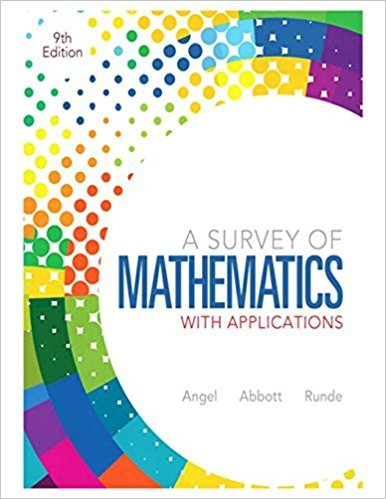×
×

# Solutions for Chapter 8.2: The Metric System## Full solutions for A Survey of Mathematics with Applications | 9th Edition

ISBN: 9780321759665Solutions for Chapter 8.2: The Metric System

Solutions for Chapter 8.2
4 5 0 305 Reviews
25
5
##### ISBN: 9780321759665

Since 115 problems in chapter 8.2: The Metric System have been answered, more than 74856 students have viewed full step-by-step solutions from this chapter. This textbook survival guide was created for the textbook: A Survey of Mathematics with Applications, edition: 9. This expansive textbook survival guide covers the following chapters and their solutions. Chapter 8.2: The Metric System includes 115 full step-by-step solutions. A Survey of Mathematics with Applications was written by and is associated to the ISBN: 9780321759665.

Key Math Terms and definitions covered in this textbook
• Big formula for n by n determinants.

Det(A) is a sum of n! terms. For each term: Multiply one entry from each row and column of A: rows in order 1, ... , nand column order given by a permutation P. Each of the n! P 's has a + or - sign.

• Circulant matrix C.

Constant diagonals wrap around as in cyclic shift S. Every circulant is Col + CIS + ... + Cn_lSn - l . Cx = convolution c * x. Eigenvectors in F.

• Complex conjugate

z = a - ib for any complex number z = a + ib. Then zz = Iz12.

• Condition number

cond(A) = c(A) = IIAIlIIA-III = amaxlamin. In Ax = b, the relative change Ilox III Ilx II is less than cond(A) times the relative change Ilob III lib II· Condition numbers measure the sensitivity of the output to change in the input.

• Cyclic shift

S. Permutation with S21 = 1, S32 = 1, ... , finally SIn = 1. Its eigenvalues are the nth roots e2lrik/n of 1; eigenvectors are columns of the Fourier matrix F.

• Dot product = Inner product x T y = XI Y 1 + ... + Xn Yn.

Complex dot product is x T Y . Perpendicular vectors have x T y = O. (AB)ij = (row i of A)T(column j of B).

• Four Fundamental Subspaces C (A), N (A), C (AT), N (AT).

Use AT for complex A.

• Free columns of A.

Columns without pivots; these are combinations of earlier columns.

• Hankel matrix H.

Constant along each antidiagonal; hij depends on i + j.

• Hilbert matrix hilb(n).

Entries HU = 1/(i + j -1) = Jd X i- 1 xj-1dx. Positive definite but extremely small Amin and large condition number: H is ill-conditioned.

• Hypercube matrix pl.

Row n + 1 counts corners, edges, faces, ... of a cube in Rn.

• Norm

IIA II. The ".e 2 norm" of A is the maximum ratio II Ax II/l1x II = O"max· Then II Ax II < IIAllllxll and IIABII < IIAIIIIBII and IIA + BII < IIAII + IIBII. Frobenius norm IIAII} = L La~. The.e 1 and.e oo norms are largest column and row sums of laij I.

• Polar decomposition A = Q H.

Orthogonal Q times positive (semi)definite H.

• Pseudoinverse A+ (Moore-Penrose inverse).

The n by m matrix that "inverts" A from column space back to row space, with N(A+) = N(AT). A+ A and AA+ are the projection matrices onto the row space and column space. Rank(A +) = rank(A).

• Saddle point of I(x}, ... ,xn ).

A point where the first derivatives of I are zero and the second derivative matrix (a2 II aXi ax j = Hessian matrix) is indefinite.

• Toeplitz matrix.

Constant down each diagonal = time-invariant (shift-invariant) filter.

• Tridiagonal matrix T: tij = 0 if Ii - j I > 1.

T- 1 has rank 1 above and below diagonal.

• Unitary matrix UH = U T = U-I.

Orthonormal columns (complex analog of Q).

• Vector space V.

Set of vectors such that all combinations cv + d w remain within V. Eight required rules are given in Section 3.1 for scalars c, d and vectors v, w.

• Vector v in Rn.

Sequence of n real numbers v = (VI, ... , Vn) = point in Rn.

×